## CodeNewbie Community is a community of 5,577 amazing newbies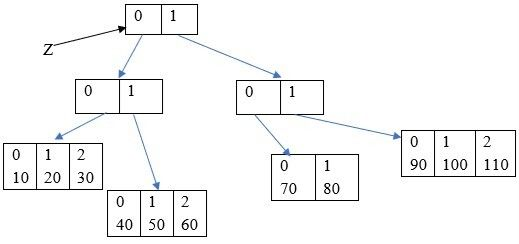Abhann

Posted on

# Arrays In Java

The Array section is spread into a three-part series because it’s quite broad and the need to understand better is efficient in this regard.

o Two-dimensional array creation:
In the first part we covered how to create a one-dimensional array, now let’s talk about the two-dimensional array creation. I will mostly use a two-dimensional array for examples, but the concepts can be applied to any multi-dimensional array in equal regard.
In this section, I will use examples as a point of demystifying the keywords and any other concepts just to create a space where the fundamentals can be clearly understood, for more understanding, try to spend enough time with the examples and also read more to expand your horizon.

In C language and C ++, a two-dimensional array is also known as a matrix.

A matrix is a structured array of data arranged in rows and columns and they are commonly written in box brackets. The matrix usage resulted into memory wastage and hence in Java the need to utilize usage brought about a different approach to multi-dimensional array creation, a concept called “Array of Arrays”.

In java, a two-dimensional array is not implemented by using the matrix approach, instead, the “array of arrays” approach is followed.
The main advantage of this approach is memory utilization will be improved.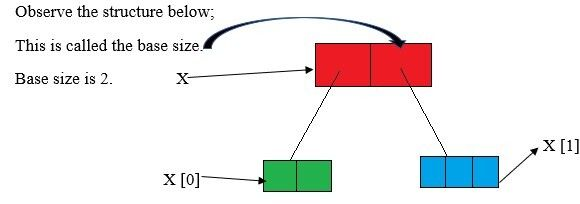As shown above, the structure represents a two-dimensional array, for the sake of a clearer understanding of the processes in the jvm(Java Virtual Machine), it’s important to try and understand the memory representation above.
X  is the first element of the base size 2, with array size two also, and the next, X  is the second element with array size three.

Further-more, in the memory, the red box is referred to as the dimension 1, and where the green and blue boxes stand is referred to as the dimension 2, and in total, they all make up the two-dimensional array structure. It is known as the Array of Arrays Concept.
Below is the statement in code: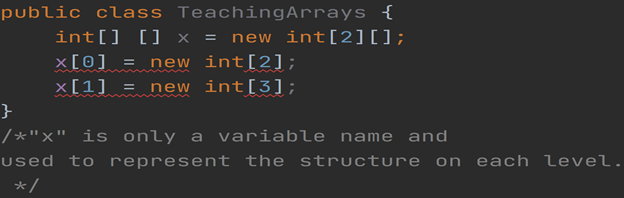In the second line of code, the second array after “” is left empty because it is not fixed (i.e. sometimes 2, and sometimes 3) as seen in the code that follows, and the structure as well, hence it is important to always specify the base size.

Now try considering the following below, and determine which of them are valid arrays;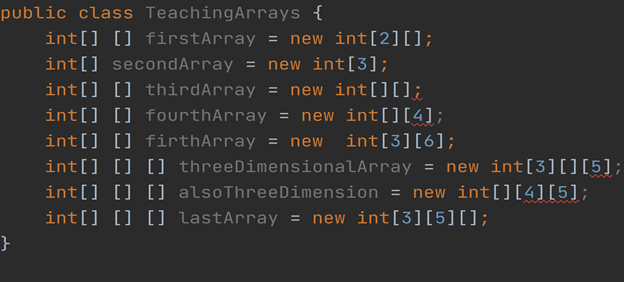o Array Initialization
Suppose: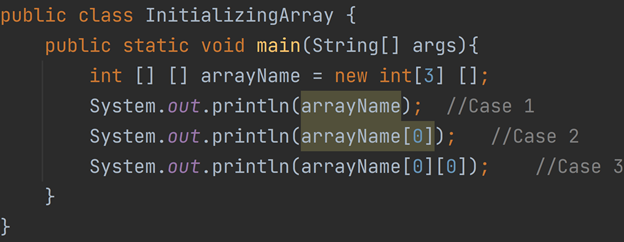The Three cases will be explained below;
First Case
Whenever we are trying to print any reference variable, internally (i.e. in the jvm), the method that will be called upon is known as the “toString” method, and its purpose by default is to return the class name, alongside it’s hashcode, which varies from P.C specifications.
Note: hashcode is in hexa-decimal form.

Second Case
Once we create an array, every array element is automatically assigned and initialized with a default value. The default value for any object reference is “null”.

Third Case
If we try to perform any operation on null, then we will get a run-time exception saying “NullPointerException”.

The following is the result at my IDE’s output for a better description of all three cases accordingly;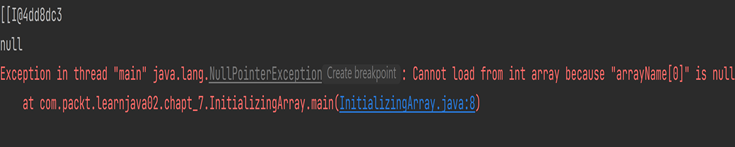o Explanation of Default Initialization
Suppose: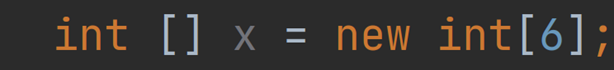As seen above, we created an array with base size 6, it follows that, every array element by default is initialized with default values which is;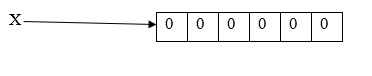The default values are the zeros “0”, now if we are not satisfied with the default values (i.e. that is, you want to make your own modifications) then happily we can override all the default values with our made-up custom values as follows: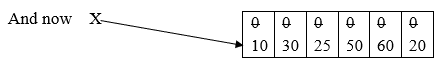• Now if we add “x” or “x[-6]”, we will get a run-time error saying: “ArrayIndexOutOfBoundsException” and this is simply because we are trying to access the array elements with an index that is out of the stated range (i.e. the range requirement is 0 – 5).

• Also, if we try to access it with a data type that isn’t compatible, for example “x[2.5]”, then we will get a compile time error saying:
Compile error: possible loss of precision.
found: double.
required: int.

• The first two invalids are known as “out of bound” and “classical mistake” respectively, and the last invalid is known as a “synthetical mistake”.

o Array Declaration, Creation and Initialization in a Single Line.
Observe below, were we subtly recap of all we’ve learnt thus far in this section:
Declaring an array;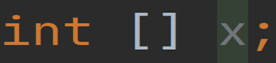Since we are all already familiar with the syntaxes, it is not considered a good programming practise to just declare, create and initialize an array on those many lines.

That many lines can be converted to a single line (i.e. A short-cut representation)

Other representation for data types, is also the same, as shown below;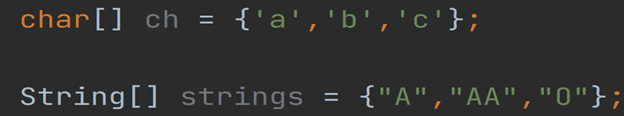We can extend this short-cut representation for multi-dimensional arrays also, as shown below;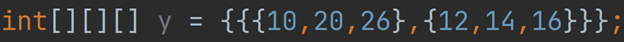The example above is a three-dimensional array element with base size 2.

Attempt the following: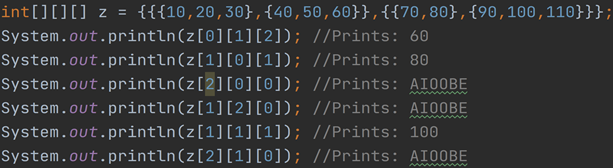The best way to answer this question is to firstly convert to a structure or memory representation as shown very much earlier, say you were asked in an interview or exam for example.
It is expressed the structure below;It makes more sense to determine the answers this way, right? all you have to do here is narrow it down to determine the required element. The “0s”, “1s” and “2s” are simply index representations.

Above all, AIOOBE is the error “ArrayIndexOutOfBoundsException”.

In conclusion, when we use the short-cut representation, it is compulsory to perform all activities in a single line, if we attempt to divide into multiple lines, then we will get a compile time error.